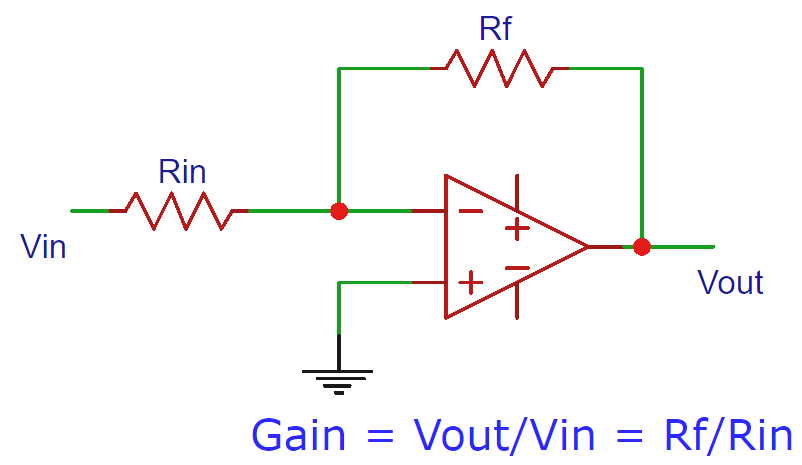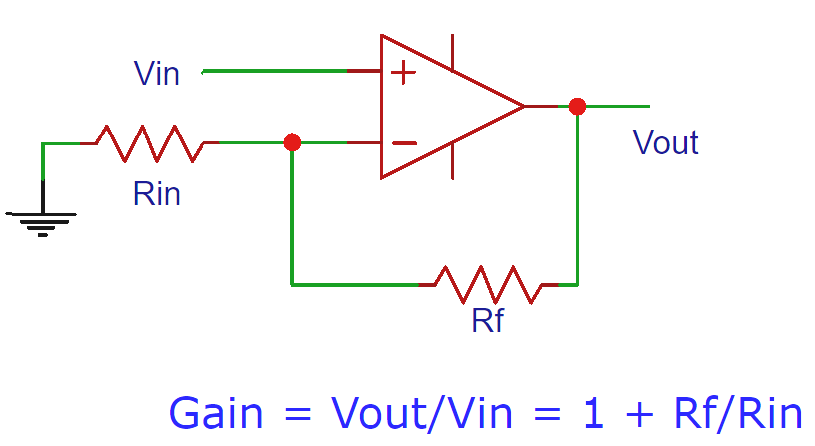# Op Amp Gain Calculator

Enter the input resistor and feedback resistor in the below op-amp calculators to calculate the voltage gain.

### Working of Inverting Op-amp Gain CalculatorInverting op-amp gain calculator calculates the gain of inverting op-amp according to the input resistor Rin and feedback resistor Rf. The gain indicates the factor by which the output voltage is amplified, i.e. it tells how many times the output voltage will be than the input voltage. The equation to calculate the gain is given below

`Gain  = Rf/Rin`

For example if the gain is 5, then the output voltage will be 5 times greater than the input voltage.

### Working of non-Inverting Op-amp Gain CalculatorThis non-inverting op-amp gain calculator calculates the gain for non-inverting op-amp according to the below equation, where Rin is the input resistor and Rf is the feedback resistor.

` Gain = 1 + Rf/Rin`